# Olympiad Test: Exponents And Powers

Test Description

## 20 Questions MCQ Test NCERT Mathematics for CAT Preparation | Olympiad Test: Exponents And Powers

Olympiad Test: Exponents And Powers for Class 8 2022 is part of NCERT Mathematics for CAT Preparation preparation. The Olympiad Test: Exponents And Powers questions and answers have been prepared according to the Class 8 exam syllabus.The Olympiad Test: Exponents And Powers MCQs are made for Class 8 2022 Exam. Find important definitions, questions, notes, meanings, examples, exercises, MCQs and online tests for Olympiad Test: Exponents And Powers below.
Solutions of Olympiad Test: Exponents And Powers questions in English are available as part of our NCERT Mathematics for CAT Preparation for Class 8 & Olympiad Test: Exponents And Powers solutions in Hindi for NCERT Mathematics for CAT Preparation course. Download more important topics, notes, lectures and mock test series for Class 8 Exam by signing up for free. Attempt Olympiad Test: Exponents And Powers | 20 questions in 20 minutes | Mock test for Class 8 preparation | Free important questions MCQ to study NCERT Mathematics for CAT Preparation for Class 8 Exam | Download free PDF with solutions
 1 Crore+ students have signed up on EduRev. Have you?
Olympiad Test: Exponents And Powers - Question 1

### What is the value of (30−40) × 5−3 ?

Detailed Solution for Olympiad Test: Exponents And Powers - Question 1

Since ao = 1 for any 'a', (30−40) × 5−3 = (1−1) × 5−3 = 0

Olympiad Test: Exponents And Powers - Question 2

### Expand 1256.249 using exponents.

Detailed Solution for Olympiad Test: Exponents And Powers - Question 2

⇒ (1 x 1000) +(2 x 100) + (5 x 10) + (6 x 1) + (2/10) + (4/100) + (9/1000) .

⇒ (1 x 103) + (2 x 102) + (5 x 101) + (6 x 100) + (2 x 10-1) + (4 x 10-2) +(9 x 10-3) (Ans)

Olympiad Test: Exponents And Powers - Question 3

### What is the solution of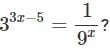Detailed Solution for Olympiad Test: Exponents And Powers - Question 3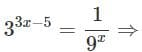33x−5 = 3−2x ⇒ 3x−5 =−2x

[When bases are equal, powers can be equated.]

⇒ 5x = 5

⇒ x = 1

Olympiad Test: Exponents And Powers - Question 4

Simplify: (−3)2×(5/3)2

Detailed Solution for Olympiad Test: Exponents And Powers - Question 4

(-3)x (5/3)= (-1)2(3)2-2(25) = 25

Olympiad Test: Exponents And Powers - Question 5

Write the expression using exponents: 89 × 89 × 89 × 89

Olympiad Test: Exponents And Powers - Question 6

Evaluate exponential expression − 25.

Olympiad Test: Exponents And Powers - Question 7

The value of 23 is _____.

Detailed Solution for Olympiad Test: Exponents And Powers - Question 7

23 = 2x2x2 = 8

Olympiad Test: Exponents And Powers - Question 8

Simplify and write in exponential form: p3×p−10

Olympiad Test: Exponents And Powers - Question 9

Very small numbers can be expressed in standard form using __________ exponents.

Olympiad Test: Exponents And Powers - Question 10

a= _______

Olympiad Test: Exponents And Powers - Question 11

The area of a square is given by the formula A = c2. What will be the total area of 5 such similar squares, if the side of a square is 8 ft?

Detailed Solution for Olympiad Test: Exponents And Powers - Question 11 We have,length of each side of a square, s = 8 ftarea of 1 square = s^2 = 8 x 8 = 64 ft^2Now, area of 5 squares = 5 x 64 = 320 ft^2
Olympiad Test: Exponents And Powers - Question 12

Evaluate the exponential expression (−n)4× (−n)2, for n = 5.

Detailed Solution for Olympiad Test: Exponents And Powers - Question 12 (-n)^4×(-n)^2for n =(-5)^4×(-5)^2 by formula if(a)^n×(a)^m=a^m+n =(-5)^4+2 =-5^6 =5×5×5×5×5×5 =15625
Olympiad Test: Exponents And Powers - Question 13

The area of a square is given by the formula A = side2. What will be the total area of 5such similar squares, if the side of a square is 7 in?

Detailed Solution for Olympiad Test: Exponents And Powers - Question 13

Side of the square is 7 inches
So area of one square is  7= 49
Total area of 5 such squares is
49×549×5 = 245 in2

Olympiad Test: Exponents And Powers - Question 14

Which of the following is the value of (4 / 5)-9 / (4 / 5)-9?

Detailed Solution for Olympiad Test: Exponents And Powers - Question 14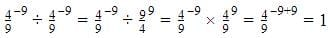Olympiad Test: Exponents And Powers - Question 15

Simplify: 25÷ 2−6

Detailed Solution for Olympiad Test: Exponents And Powers - Question 15

25/2-6 = 25-(-6) = 211

Olympiad Test: Exponents And Powers - Question 16

Simplify:  ((1/3)-2 - (1/2)-3)/ (1/4)-2

Olympiad Test: Exponents And Powers - Question 17

Find the value of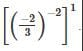Olympiad Test: Exponents And Powers - Question 18

Find the value of the expression a2 for a = 10.

Detailed Solution for Olympiad Test: Exponents And Powers - Question 18

a = 10, a2 = 102
10x10 =100

Olympiad Test: Exponents And Powers - Question 19

Evaluate: - 73

Olympiad Test: Exponents And Powers - Question 20

Find the multiplicative inverse of 7−2.

Detailed Solution for Olympiad Test: Exponents And Powers - Question 20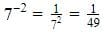## NCERT Mathematics for CAT Preparation

276 docs|149 tests
 Use Code STAYHOME200 and get INR 200 additional OFF Use Coupon Code